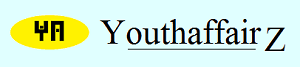﻿ Youthaffairz | Teaser: Festival shoppingNovember 2015

# Solution

Let's suppose I started off with "x" rupees in my pocket.

I visit the first shop and spend 100 rupees more that half of "x"; this means I spend (x/2 + 100) rupees in the first shop. So, I am left with x - (x/2 + 100); thus, I am left with (x - 200)/2.

In the second shop I spend 100 rupees more than half of what I have left. So, I spend [(x - 200)/4 + 100]. On coming out of the second shop I am left with (x - 200)/2 - [(x - 200)/4 + 100]. Thus, I am left with (x - 600)/4.

In the third shop I again spend 100 rupees more than half of what I have left; so, I spend [(x - 600)/8 + 100]. On coming out of the third shop I am left with [(x - 600)/4] - [(x - 600)/8 + 100]. So, I am left with (x - 1400)/8.

In the fourth shop I spend 100 rupees more than half of what I have left; so, I spend [(x - 1400)/16 + 100]. On coming out of the fourth shop I am left with (x - 1400)/8 - [(x - 1400)/16 + 100]. So, I am left with (x - 3000)/16.

In the fifth shop I spend 100 rupees more than half of what I have left; so, I spend [(x - 3000)/32 + 100]. On coming out of the fourth shop I am left with (x - 3000)/16 - [(x - 3000)/32 + 100]. So, I am left with (x - 6200)/32.

But on coming out of the fifth shop I am left with no money. So, (x - 6200)/32 = 0. Thus, x - 6200 = 0. So, x = 6200. I, therefore, started with Rs 6200 in my pocket.

Let's work backwards to see if I got the answer right. I have 6200 rupees and enter the first shop. I spend (6200/2 + 100). Thus, I spend 3200. I am left with 3000.

In the second shop I spend 3000/2 + 100 = 1600. I am left with 1400.

In the third shop I spend 1400/2 + 100 = 800. I am left with 600.

In the fourth shop I spend 600/2 + 100 = 400. I am left with 200.

In the fifth shop I spend 200/2 + 100 = 200. I am left with no money.

• Union Public Service Commission - www.upsc.gov.in
• IIT-Kharagpur - www.iitkgp.ac.in
• Indian Statistical Institute - www.isical.ac.in
• Indian Institute of Technology Madras - www.iitm.ac.in
• Indian Institute of Management, Ahmedabad - www.iimahd.ernet.in
• Indian Institute of Mass Commission - www.iimc.nic.in
• IIT Bombay - www.iitb.ac.in